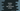# Python program to print a half pyramid in star## Python program to print half pyramid in star:

This program will show you how to print a pyramid in star * using python. We choose *, but we can always go for any other character that we want. We will learn different types of Pyramid patterns to print in this post.

### Pattern 1: Half pyramid pattern:

Let’s create one half pyramid first :

``````def print_pyramid(size):
for row in range(0, size):
for col in range(0, row+1):
print("*", end=" ")
print("")

size = int(input("Enter the size of the Pyramid : "))
print_pyramid(size)``````

Here, we are taking the size of the pyramid as input and printing the pyramid. For example:

``````Enter the size of the Pyramid : 6
*
* *
* * *
* * * *
* * * * *
* * * * * * ``````
• print_pyramid method is responsible for printing the pyramid.
• It takes the size of the pyramid as parameter and prints it out.
• We have two for loops in this method. The outer loop is used to indicate the rows for the pyramid.
• The inner loop is to print the * i.e. the columns of the triangle.
• The outer loop runs from 0 to size, i.e. if we are passing 5 as size, it will run for 5 times. The inner loop runs for row+1 times where row is the current outer loop value.

### Patern 2: Inverted right angled triangle:

We can also print one inverted right angled triangle by taking the size as input. The logic is difficult than the previous one. It will look as like:

``````    *
**
***
****
*****``````

Here, we need to print blank spaces before printing the star. For this pyramid:

• The height is 5
• For the first line, we are printing 4 blank spaces and one *.
• For the second line, we are printing 3 blank spaces and two *.
• i.e. for nth line, we are printing size - n number of blank spaces and n number of *.

It looks as like below if we write in code:

``````def print_pyramid(size):
for row in range(0, size):
for i in range(0, size - row - 1):
print(' ', end='')
for i in range(0, row + 1):
print('*', end='')
print('')

size = int(input('Enter the size of the Pyramid : '))
print_pyramid(size)``````

If you execute this program, it will print outputs as like below:

``````Enter the size of the Pyramid : 10
*
**
***
****
*****
******
*******
********
*********
**********``````

You can give any size to print a half pyramid.